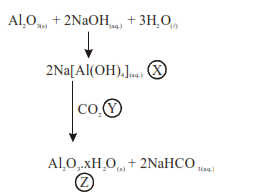# Al2O3 was leached with alkali to get X. The solution of X on passing of gas Y, forms Z. X, Y and Z respectively are :`
Question:

$\mathrm{Al}_{2} \mathrm{O}_{3}$ was leached with alkali to get $\mathrm{X}$. The solution of $\mathrm{X}$ on passing of gas $\mathrm{Y}$, forms $\mathrm{Z}$. X, $Y$ and $Z$ respectively are :

1. $\mathrm{X}=\mathrm{Na}\left[\mathrm{Al}(\mathrm{OH})_{4}\right], \mathrm{Y}=\mathrm{SO}_{2}, \mathrm{Z}=\mathrm{Al}_{2} \mathrm{O}_{3}$

2. $\mathrm{X}=\mathrm{Na}\left[\mathrm{Al}(\mathrm{OH})_{4}\right], \mathrm{Y}=\mathrm{CO}_{2}, \mathrm{Z}=\mathrm{Al}_{2} \mathrm{O}_{3} \cdot \mathrm{XH}_{2} \mathrm{O}$

3. $\mathrm{X}=\mathrm{Al}(\mathrm{OH})_{3}, \mathrm{Y}=\mathrm{CO}_{2}, \mathrm{Z}=\mathrm{Al}_{2} \mathrm{O}_{3}$

4. $\mathrm{X}=\mathrm{Al}(\mathrm{OH})_{3}, \mathrm{Y}=\mathrm{SO}_{2}, \mathrm{Z}=\mathrm{Al}_{2} \mathrm{O}_{3} \cdot \mathrm{xH}_{2} \mathrm{O}$

Correct Option: , 2

Solution:So

$\mathrm{X}: \mathrm{Na}\left[\mathrm{Al}(\mathrm{OH})_{4}\right]$

$\mathrm{Y}: \mathrm{CO}_{2}$

$\mathrm{Z}: \mathrm{Al}_{2} \mathrm{O}_{3} \cdot \mathrm{xH}_{2} \mathrm{O}$